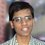# Inequality on $e$

$\Large{ e < \left( \dfrac{(n+1)^{2n+1}}{(n!)^2} \right)^\frac{1}{2n} < e^\alpha }$

Let $n$ be a positive integer. If $\alpha = 1 + \dfrac{1}{12(n+1)}$, prove that the above expression holds.Note by Satyajit Mohanty
4 years, 11 months ago

This discussion board is a place to discuss our Daily Challenges and the math and science related to those challenges. Explanations are more than just a solution — they should explain the steps and thinking strategies that you used to obtain the solution. Comments should further the discussion of math and science.

When posting on Brilliant:

• Use the emojis to react to an explanation, whether you're congratulating a job well done , or just really confused .
• Ask specific questions about the challenge or the steps in somebody's explanation. Well-posed questions can add a lot to the discussion, but posting "I don't understand!" doesn't help anyone.
• Try to contribute something new to the discussion, whether it is an extension, generalization or other idea related to the challenge.

MarkdownAppears as
*italics* or _italics_ italics
**bold** or __bold__ bold
- bulleted- list
• bulleted
• list
1. numbered2. list
1. numbered
2. list
Note: you must add a full line of space before and after lists for them to show up correctly
paragraph 1paragraph 2

paragraph 1

paragraph 2

[example link](https://brilliant.org)example link
> This is a quote
This is a quote
    # I indented these lines
# 4 spaces, and now they show
# up as a code block.

print "hello world"
# I indented these lines
# 4 spaces, and now they show
# up as a code block.

print "hello world"
MathAppears as
Remember to wrap math in $$ ... $$ or $ ... $ to ensure proper formatting.
2 \times 3 $2 \times 3$
2^{34} $2^{34}$
a_{i-1} $a_{i-1}$
\frac{2}{3} $\frac{2}{3}$
\sqrt{2} $\sqrt{2}$
\sum_{i=1}^3 $\sum_{i=1}^3$
\sin \theta $\sin \theta$
\boxed{123} $\boxed{123}$

Sort by:

@Satyajit Mohanty What method did you use to solve this question? Also, one of your questions has totally stumped me. Does it have a nice closed form, or do we have to evaluate it numerically? Can you post a solution to that question too? Thanks.

- 4 years, 10 months ago

And can you please also tell what method did you use for this inequality problem or at least give a hint?

- 4 years, 10 months ago

@Satyajit Mohanty Sorry to disturb you again, but can you please add a solution to your 300 followers problem?

- 4 years, 10 months ago

@Samuel Jones - I've added the solution to the problem 300 Followers Problem - Polynomial Differential Reciprocal Summations!. Please check it.

- 4 years, 10 months ago